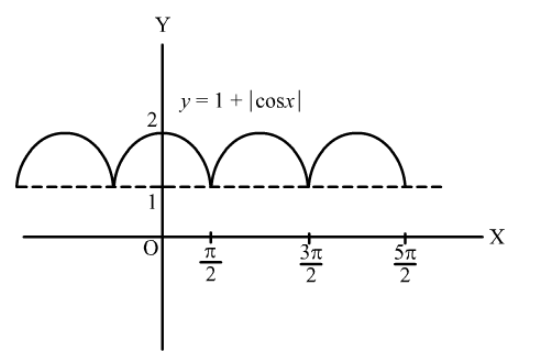# The function f (x) = 1 + |cos x| is

Question:

The function $f(x)=1+|\cos x|$ is

(a) continuous no where

(b) continuous everywhere

(c) not differentiable at $x=0$

(d) not differentiable at $x=n \pi, n \in Z$

Solution:

(b) continuous everywhere

Graph of the function $f(x)=1+|\cos x|$ is as shown below:From the graph, we can see that $f(x)$ is everywhere continuous but not differentiable at $x=(2 n+1) \frac{\pi}{2}, n \in Z$